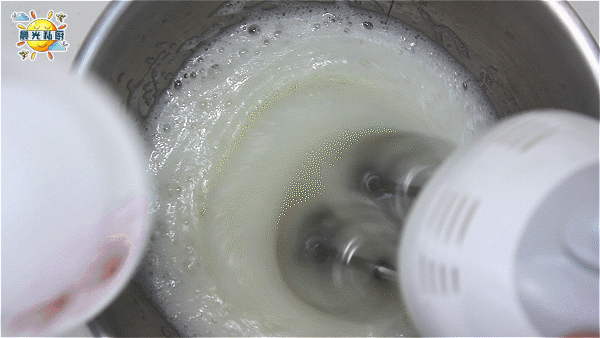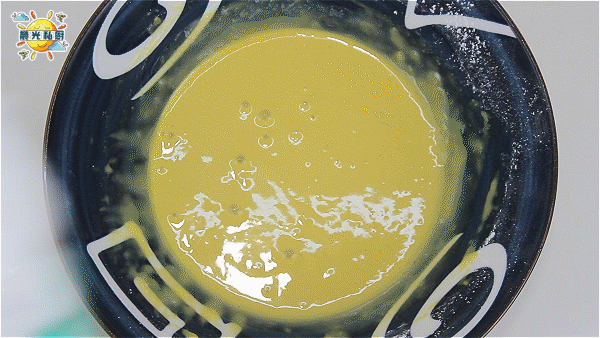# 顺滑松软的波士顿派，从蛋黄的乳化到蛋白的干性发泡一次性教给你2021-05-09 19:24

195112 0 0# 食材清单

• 低筋面粉 × 60克
• 鸡蛋 × 3个（带壳约重60克/个）
• 全脂纯牛奶 × 40克
• 植物油 × 40克
• 香草精 × 10克
• 细砂糖 × 50克
• 柠檬汁 × 5克
• 糖粉 × 适量

• 动物性淡奶油 × 200克
• 细砂糖 × 20克# 详细烹饪步骤

-= Step 01 =–-= Step 02 =–-= Step 03 =–-= Step 04 =–-= Step 05 =–-= Step 06 =–-= Step 07 =–-= Step 08 =–-= Step 09 =–-= Step 10 =–-= Step 11 =–-= Step 12 =–-= Step 13 =–-= Step 14 =–-= Step 15 =–-= Step 16 =–# 要点与小贴士

-= Tips and Point 01 =–-= Tips and Point 02 =–-= Tips and Point 03 =–# 晨光碎碎念1 20天前
2 6 25天前
4 9 6天前